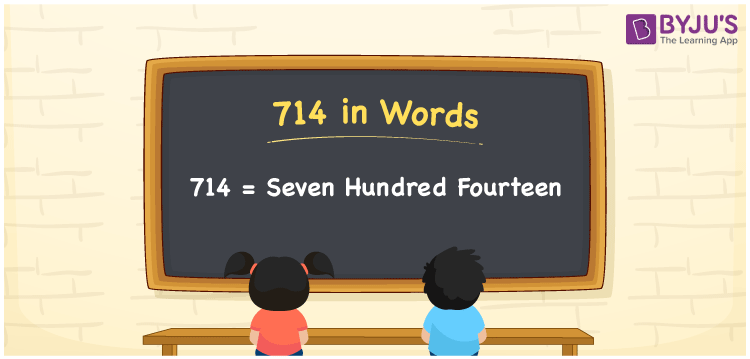# 714 in Words

We can write 714 in words as Seven hundred fourteen. If you purchased a box of sweets at Rs. 714, then you can say, “I purchased a box of sweets at Seven hundred fourteen rupees”. From this statement, we can say that Seven hundred fourteen is the number name for 714. In this article, you will learn how to write the cardinal number 714 in word form using a simple technique.

 714 in words Seven hundred fourteen Seven hundred fourteen in Numbers 714

## 714 in English Words

We generally use the English alphabet to express numbers in words. So, we can spell 714 in English words as “Seven hundred fourteen”.## How to Write 714 in Words?

To convert the number 714 into words, we need to create a place value chart with three columns since the number 714 contains three digits. This can be done as follows.

 Hundreds Tens Ones 7 1 4

Here, ones = 4, tens = 1, hundreds = 7

By expanding the above digits based on their place values, we get;

7 × Hundred + 1 × Ten + 4 × One

= 7 × 100 + 1 × 10 + 4 × 1

= 700 + 10 + 4

= Seven hundred + Ten + Four

= Seven hundred fourteen {since ten + four = fourteen}

Hence, 714 in words = Seven hundred fourteen

We know that 714 is a natural number that precedes 715 and succeeds 713.

714 in words – Seven hundred fourteen

Is 714 an odd number? – No

Is 714 an even number? – Yes

Is 714 a prime number? – No

Is 714 a composite number? – Yes

Is 714 a perfect square number? – No

Is 714 a perfect cube number? – No

## Frequently Asked Questions on 714 in Words

Q1

### How do you write 714 in words?

We can write the number 714 in English words as Seven hundred fourteen.
Q2

### What number is Seven hundred fourteen in words?

The number 714 is expressed as Seven hundred fourteen in words. Therefore, 714 = Seven hundred fourteen.
Q3

### How to write Rs. 714 in words on a cheque?

On a cheque, an amount of Rs. 714 can be written in words as “Seven hundred fourteen rupees only”.